# Hackpact

Random explorations through noise
emoc, September 2009

## september 1 : basic noise

Some noise output by ChucK, using the Noise ugen, and CNoise (Colored Noise?), an undocumented ugen (see chuck forum post) that generates different types of noise but needs a fix!( * )

```Noise n => dac;
.1 => n.gain;
while( 100::ms => now ) {}```
```CNoise n => dac;
1 => n.gain;
while( 100::ms => now ) {}```
Ten seconds of white noise...

## september 2 : subnoise

Playing with SubNoise ugen, a subsampled noise generator.

```SubNoise sn => blackhole;
Step s=>dac;
.5 => s.gain;
while(100::samp=>now){
sn.last() => s.next;
}```

Here is the waveform : looks like a cityscape in defender...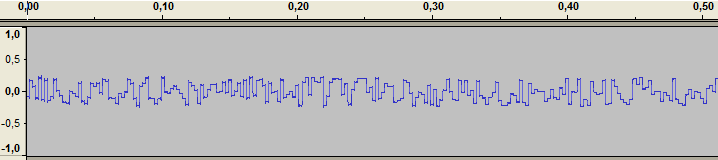Mouse controlled! Move the mouse to change the step length : slow on X, faster on Y. Pressing a button resets the step length to 1/44100 at the mouse position. It could have been achieved by classic random numbers...

subnoise+mouse.ck

```0 => int deviceNum;
Hid hi;
HidMsg msg;
if( !hi.openMouse( deviceNum ) ) me.exit();

SubNoise sn => blackhole;
Step s => dac;
.5 => s.gain;
1::samp => dur d;

fun void sound() {
while(d => now) {
sn.last() => s.next;
}
}

spork ~ sound();

while(true) {
hi => now;
while( hi.recv( msg ) ) {
if( msg.isMouseMotion() ) {
if( msg.deltaX ) {
d + (msg.deltaX * .1::samp) => d;
if (d < 1::samp) 1::samp => d;
} else if( msg.deltaY ) {
d + (msg.deltaY * 2::samp) => d;
if (d < 1::samp) 1::samp => d;
}
} else if( msg.isButtonDown() ) {
1::samp => d;
}
}
}```
Sounds from the last ChucK script

## september 3 : making an inventory

Different flavors of noise : besides famous white (= Johnson noise) and pink noises (also called 1/f noise or flicker noise), searching the web reveals numerous other noises. The rainbow family, arranged by spectral density : red noise, blue noise / azure noise, violet noise / purple noise, grey noise, red noise, orange noise, green noise, and the mysterious black noise (1). Other noises include : brownian noise (also known as brown noise), gaussian noise, flip-bit noise, xor and fbm (from chuck CNoise ugen), Perlin noise for procedural textures, simplex noise, wavelet noise, gradient noise or value noise (2), popcorn noise, thermal noise. All those noises don't relate to audio, but to other fields : image processing, communications, physics, mathematical chaos theory (3).
Some elements from this words cloud could evaporate through further advances, it could be enlarged too...

References :
(1) http://en.wikipedia.org/wiki/Colors_of_noise
(2) http://en.wikipedia.org/wiki/Perlin_noise
(3) http://www.rane.com/par-n.html#noise_color

## september 4 : line noise

Trying another method : instead of selecting a random value for each sample, I'll try drawing a line segment between two random values at a fixed interval, between those two points each sample value is computed by interpolation. Two methods of interpolation tested : basic linear and cosine interpolation, there are many other methods that I'll perhaps try later, easing / tweening functions could give nice results too!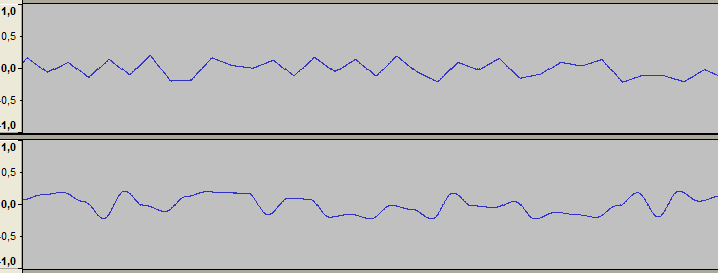10 seconds of line noise with linear interpolation (segment = 34 samples)

10 seconds of line noise with cosine interpolation (segment = 34 samples)

Mouse controls :
- move the mouse fast from left to right to modify segment length
- left button resets segment size to 50
- right button switch between linear & cosine interpolation

line_noise_interpolation+mouse.ck

```// line noise with interpolation - http://emoc.org/hackpact/ - sep 2009

0 => int deviceNum;
Hid hi;
HidMsg msg;
if( !hi.openMouse( deviceNum ) ) me.exit();

Step s => dac;
.5 => s.gain;
50 => int length; // length of a segment
0 => int i;
Std.rand2f(-1,1) => float n;
Std.rand2f(-1,1) => float n1;
1::samp => dur d;
0 => int interpolation;

fun void sound() {
while (1::samp => now) {
if (i < length) {
if (interpolation == 0) {
cosine_interpolation(n, n1, i\$float/length) => s.next;
} else {
linear_interpolation(n, n1, i\$float/length) => s.next;
}
i++;
} else {
0 => i;
n1 => n;
Std.rand2f(-1,1) => n1;
}
}
}

fun float cosine_interpolation(float a, float b, float x) {
(1 - Math.cos(x * 3.1415927)) * .5 => float f;
return a*(1-f) + b*f;
}

fun float linear_interpolation(float a, float b, float x) {
return a*(1-x) + b*x;
}

fun void switch_interpolation() {
if (interpolation == 0) {
1 => interpolation;
<<<"linear interpolation">>>;
} else {
0 => interpolation;
<<<"cosine interpolation">>>;
}
}

spork ~ sound();

while(true) {
hi => now;
while( hi.recv( msg ) ) {
if( msg.isMouseMotion() ) {
if( msg.deltaX ) {
// move the mouse fast from left to right to modify segment length
length + Math.sgn(msg.deltaX/3)\$int => length;
if (length < 5) 5 => length;
if (length > 100) 100 => length;
<<<length, msg.deltaX>>>;
}
} else if( msg.isButtonDown() ) {
// left button resets segment size
// right button switch between linear & cosine interpolation
if (msg.which == 0) 50 => length;
if (msg.which == 1) switch_interpolation();
}
}
}```

## september 5 : rumbling and frying

Introducing more noise, segment size changes for each segment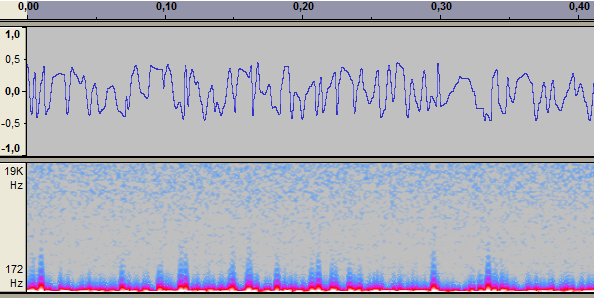10 seconds noise with random segments

line_random_segment.ck

```Step s => dac;
1 => s.gain;
50 => int length; // length of a segment
0 => int i;
Std.rand2f(-1,1) => float n;
Std.rand2f(-1,1) => float n1;

while (1::samp => now) {
if (i < length) {
cosine_interpolation(n, n1, i\$float/length) => s.next;
i++;
} else {
0 => i;
Std.rand2(40,200) => length; // reset length
n1 => n;
Std.rand2f(-1,1) => n1;
}
}

fun float cosine_interpolation(float a, float b, float x) {
(1 - Math.cos(x * 3.1415927)) * .5 => float f;
return a*(1-f) + b*f;
}
```

Something completely different, chunk of sine waves are pasted together, n cycles for each frequency :

sine suite

random_sine_suite.ck

```Step s => dac;
.4 => s.gain;

0 => int i;
0 => int re;
15 => int n; // number of repetitions

Std.rand2f(80, 10000) => float freq;
(44100 / freq)\$int => float steps;

while (1::samp => now) {
if (i < steps) {
Math.sin((i / steps) * Math.PI * 2) => s.next;
i++;
} else {
0 => i;
if (re > n) {
0 => re;
Std.rand2f(80, 10000) =>  freq;
(44100 / freq)\$int =>  steps;
} else re++;
}
}```

Same script with interactive mouse control, moving mouse from left to right changes the times each wave repeats.

random_sine_suite+mouse.ck

## september 6 : back to colors

In his tutorial on synthesizing pouring liquid sounds, Andy Farnell / Obiwannabe gives a clear explanation of noise colours and distribution in the spectrum. He puts forward a pure-data patch to generate colored noise from a noise source, some low-pass and high-pass filters. I tried to translate this to chuck, but I'm not sure of the results because I had to tweak gain values to obtain a correct signal, need to dive a little more into filters to understand this. (Chuck filters HPF and LPF are 2nd order butterworth filters, don't know which type for pd).(Picture taken from tea tutorial by Andy Farnell)

green noise

red noise

green_noise.ck

```// green noise

HPF f1;
LPF f2;

Noise n => f1 => f1 => f1 => f1 => f2 => f2 => f2 => f2 => Gain g => dac;

3000 => f1.freq;
7000 => f2.freq;

.3 => n.gain;
.3 => f1.gain;
.3 => f2.gain;
50 => g.gain;

while (1) {
1::second => now;
}```
red_noise.ck

```// red noise

HPF f1;
LPF f2;

Noise n => f1 => f1 => f1 => f1 => f2 => f2 => f2 => f2 => Gain g => dac;

250 => f1.freq;
500 => f2.freq;

.5 => n.gain;
.5 => f1.gain;
.5 => f2.gain;
20 => g.gain;

while (1) {
1::second => now;
}```
pink_noise.ck

```// pink noise

HPF f1;
LPF f2;

Noise n => f1 => f1 => f1 => f1 => f2 => f2 => f2 => f2 => dac;

15000 => f1.freq;
20000 => f2.freq;

50 => n.gain;

while (1) {
1::second => now;
}```

## september 8 : from sin to noise

First experiments with F.I.S. (Functional Iteration Synthesis), an audio synthesis method described by Agostino Di Scipio. Audio synthesis is done through iterated nonlinear functions : F.I.S. (Functional Iteration Synthesis) pdf

Automatic ride :
fis_base.ck

```Step s => dac;
.5 => s.gain;
float n,r,o;
4 => int iterations;

while(samp=>now){
Math.cos(n*.0001)+3 => r;
n => o;
for(int i;i<iterations;i++) Math.sin(o*r) => o;
o => s.next;
n+.5 => n;
}
```
fis_base.ck

Trying an interactive exploration :
- move mouse left & right to modify r
- move up & down to modify velocity
- left button to ++ iterations
- right button to -- iterations

fis+mouse.ck

```// Functional Iteration Synthesis - http://emoc.org/hackpact/ - sep 2009

0 => int deviceNum;
Hid hi;
HidMsg msg;
if( !hi.openMouse( deviceNum ) ) me.exit();

Step s => dac;
.5 => s.gain;

5 => int iterations;
- Math.PI => float n;       // current sample
3 => float r;               // parameter of function
float o;                    // current sample value
.00001 => float velocity;    // orbital velocity

fun void sound() {
while (1::samp => now) {
n => o;
// f(n) = sin (r * n), iterated {iterations} times
for(int i; i <= iterations; i++) Math.sin(o*r)=>o;
o => s.next;
n + velocity => n;
if (n > Math.PI / 2) - Math.PI => n;
}
}

spork ~ sound();

while(true) {
hi => now;
while( hi.recv( msg ) ) {
if( msg.isMouseMotion() ) {
if( msg.deltaX ) {

// move mouse left & right to modify r
r + msg.deltaX\$float/500 => r;
if (r < 2) 2 => r;
if (r > 4) 4 => r;
<<<"r", r>>>;

} else if ( msg.deltaY ) {

// move up & down to modify velocity
velocity + msg.deltaY\$float/50000 => velocity;
if (velocity < 0) 0 => velocity;
<<<"velocity : ", velocity>>>;

}
} else if( msg.isButtonDown() ) {

// left button to ++ iterations
// right button to -- iterations
if (msg.which == 0)  iterations++;
if (msg.which == 1)  iterations--;
if (iterations < 1) 1 => iterations;
if (iterations > 13) 13 => iterations;
<<<iterations>>>;

}
}
}```

pause();

## september 27 : Perlin noise

Some visual explorations of 2D Perlin noise made with processing (Play with the applet online). Ken Perlin's algorithm creates pseudo-random noise used in procedural textures for computer graphics (see Perlin noise for a good explanation of the basics.)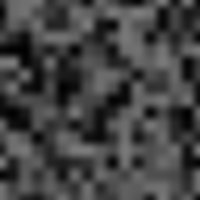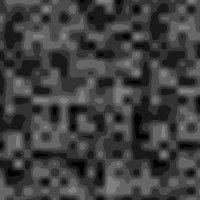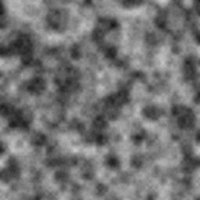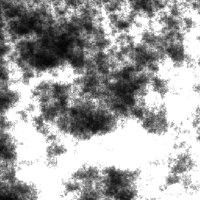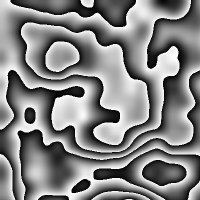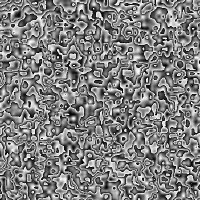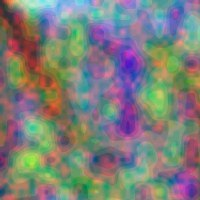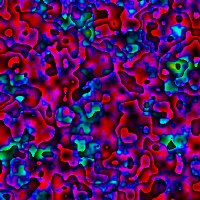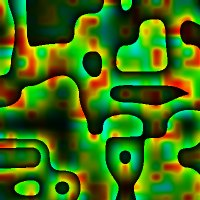## september 28 : Nature noise

Some steps outside to find some noisy noises, accidentaly found some concrete filtering of water flow ...

more relaxing waves of waves
not really noise...

## september 30: elastic noises

Taking a look back at chuck scripts of september 5th, and adding some elasticity and friction...

pendulum.ck
elasticity.ck
elasticity+mouse.ck

pendulum.ck

elasticity.ck

elasticity+mouse.ck

```// move mouse left & right to modify elasticity
// move up & down to modify friction
// left button to send a new impulse

0 => int deviceNum;
Hid hi;
HidMsg msg;
if( !hi.openMouse( deviceNum ) ) me.exit();

Step s => dac;
.5 => s.gain;

float vx, ex;
200 => float x;
.05 => float elasticity;
.005 => float friction;

x\$int => int length;
0 => int i;

-1 => float n;

fun void sound() {
while (1::samp => now) {

if (i < length) {
cosine_interpolation(n, -n, i\$float/length) => s.next;
i++;
} else {
0 => i;

elasticity * (x - 100) => ex;
(1 - friction) * (ex + vx) => vx;
x - vx => x;
x\$int => length;

-n => n;
}
}
}

fun float cosine_interpolation(float a, float b, float x) {
(1 - Math.cos(x * 3.1415927)) * .5 => float f;
return a*(1-f) + b*f;
}

spork ~ sound();

while(true) {
hi => now;
while( hi.recv( msg ) ) {
if( msg.isMouseMotion() ) {
if( msg.deltaX ) {

elasticity + msg.deltaX\$float/1000 => elasticity;
if (elasticity < .005) .005 => elasticity;
if (elasticity > 1) 1 => elasticity;
<<<"elasticity", elasticity>>>;

} else if ( msg.deltaY ) {

friction + msg.deltaY\$float/2000 => friction;
if (friction < .005) .005 => friction;
if (friction > .05) .05 => friction;
<<<"friction : ", friction>>>;

}
} else if( msg.isButtonDown() ) {

if (msg.which == 0)  300 => x;

}
}
}```

## september 30+1: Listening to Perlin

Hackpact is over! But I have some more ideas to try. Here is a picture made using a 1-dimension Perlin noise algorithm from scratch (borrowing code from Hugo Elias), and a processing applet (online version here), varying the steps increment in random noise. Full image : 7858 x 400, 1 Mo jpeg.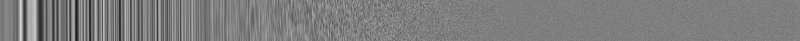Interactive audio exploration of Perlin noise. Take a ride! Modulo mode, by repeating random patterns, gives great sci-fi rollercoaster sounds.

Perlin exploration with perlin_noise+mouse.ck
perlin_noise+mouse.ck

## september 30+2: Crash Disk

My 2 disks software raid system failed today... Intel software raid on windows is crappy, don't do it. I'll keep ideas for later, post-hackpact experiments...